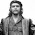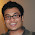### Original Problem : ATM Money Withdrawal Puzzle

Source: One of the very few original problems on the blog. Remember 'Mathematics of Housie'? Please share if you like!

Problem:
Assumptions:
a) I have infinite money in my account
b) The daily limit of amount of money that can be withdrawn from an ATM is finite
c) You can login into an ATM Machine only once a day
d) If you login into the machine and enter a large number to withdraw, you will not get anything. And hence, you will not be able to withdraw anything from the ATM for the day.
e) I do not know what the daily limit is.

What strategy should I choose so that I can withdraw N rupees in minimum number of days?

Of course, you can do it in N days (withdrawing only one rupee daily)
you could do it in N-limit + ceiling(N/limit)-1 days (check N, check N-1, .. check limit. Once you know the limit, and you have already withdrawn 'limit' rupees, you will take ceiling ((N-limit)/limit) days more.

Can you do better?

Update: (19-07-2012)
This is essentially an open ended question, until of course we get the proof that a solution is optimal.

1.using binary search algorithm to find the limit ........ so we can find the limit in log2(n) ......... after finding the limit we can withdraw the sum in ceiling((n-limit)/limit) additional days ...........so altogether we will require log2(n)+ceiling((n-limit)/limit) days............

2.I could think of a strategy that looks slightly better than the ones described in your post:
1. check 1, check 2, check 4,...., till you are refused by the ATM machine. Note that you can do it for (log limit +1) days. By that time you would have accumulated 2*limit - 1 rupees. For the remaining rupees N-2*limit + 1, draw at the rate last accepted by the ATM. That'll take you at the most 2*(N-2*limit+1)/limit days. So in total it takes you (log limit +1) + 2*(N-2*limit+1)/limit days. logarithms are to the base 2. For moderate value of limit, this seems to be a little better...The idea is to use binary search to know the limit. From there on you can draw money at the rate of limit per day.

3.I could think of a strategy that looks slightly better than the ones described in your post:
1. check 1, check 2, check 4,...., till you are refused by the ATM machine. Note that you can do it for (log limit +1) days. By that time you would have accumulated 2*limit - 1 rupees. For the remaining rupees N-2*limit + 1, draw at the rate last accepted by the ATM. That'll take you at the most 2*(N-2*limit+1)/limit days. So in total it takes you (log limit +1) + 2*(N-2*limit+1)/limit days. logarithms are to the base 2. For moderate value of limit, this seems to be a little better...The idea is to use binary search to know the limit. From there on you can draw money at the rate of limit per day.

4.One of the ways, in which the number of trials can be reduced is to use binary search for the limit, instead of linear search, i.e., In 1st attempt, try to take out N/2, if it fails, try N/4, otherwise try N/2 again and you are done. This method will give better result than the two methods described in the problem.

5.first day i withdraw n/2 rupees.if i get it then i will increase the amount by n/4 else decrease it by n/4.basically i will use binary search technique.

6.Call the limit 'l'. Call N/l as x. Obviously, x is the best we can do, so the question really is how well we can approach x in the worst case. In the case of checking 1, 2, 4, etc. it is hard to get good constant factors over x without finding out l pretty well (eg. if we find only highest power of 2 that does not exceed l, we can at best get a factor of 2x), so the binary search to find the limit seems to make more sense. In this case, suppose we find l completely first. Worst case is that l = N/(2^a) + 1 for some a. Here we will find the value of l after a (to find first power of 2 after dividing by which we can withdraw) + log (N/2^a)) (to query values between this and its double to find where l lies) queries = logN queries, and will only have removed 2l-1 from N after doing all this. Time taken here would be logN + (N-2l+1)/l, which is roughly logN + x - 2. This is quite good in terms of x, when log N is much smaller than x. Only problem is where log N is much more than x. However, this is easily dealt with. For log N to be more than x, l would have to exceed N/logN. While doing the binary search, if the first point where we are actually able to withdraw is more than this, then don't waste time trying to find exact value of l. Instead, use what we have already found and withdraw all the money using that limit. Of course, total number of days is limited by logN here, but even in terms of x, we have a limit of 2x for even these 'bad' cases.

7.For the other way with 1,a,a^2 etc.:

check N/1,N/a,N/a^2, etc. until you reach a point where the withdrawal works.

let N/a^(k+1) <= l < N/a^k.

Once you get this N/a^(k+1) (call this l'), just use this instead of finding out l exactly.

Days required, D = (k + 1) + N/l' - 1 = k + N/l'.

l/l' <= a. So N/l' <= Na/l = ax.

So D <= logx/loga + ax.

a can be taken as any arbitrarily small constant (strictly more than 1 of course, say 1 + epsilon), for constant a the second term clearly dominates.

So we can come arbitrarily close to a factor of 1 in x.

8.I think the first task is to find the limit which can be found in log2(N) trials. It is also true that after this many trial I will already have at least limit money already with me.
For an example let N=100
limit = 10
1st try = 50(NS - No Success)
2nd - 25(NS)
3rd - 12(NS)
4th - 6(S- Success)
5th - 9(S)
6th - 11(NS)
7th - 10(S)
In 7 trials I know the limit and also I have 25/- already.
(100-25)/10 ~ 8 trials.
So, 15 trials.
No. of trials = log2(N)+ (N-limit)/limit.
Also, let a is the no of trials required, then a(a+1)/2 = N is the equation to be solved for the solution. a = (sqrt(1+8N)-1)/2

9.I really appreciate the kind of topics you post here. Thanks for sharing information that is actually helpful for me.

10.Interesting solutions. Thanks for providing better than mine. Can we prove which one is the optimal?

11.Let limit be l, amount to be withdrawn be N, N/l is say x and as it happens in most cases, l is greater than sqrt(N)

My solution: N - l + ceiling(x) -1

Solution by Nick:
log2(N) + ceiling(x-1)
[Nick's solution: using binary search algorithm to find the limit. So we can find the limit in log2(N). After finding the limit we can withdraw the sum in ceiling((N-limit)/limit) additional days. So altogether we will require log2(N) + ceiling((N-limit)/limit) days]

Solution by bose's diary:
log2(limit) +2*x-3
[Check 1, Check 2, Check 4, and so on, till you are refused by ATM Machine. So, in the worst case, you would have drawn "limit" amount and you would take log2 (limit/2) days.
The rest would be withdrawn in the worst case at limit/2. So, number of days: log2 (limit/2) +(N-limit)*2/limit = log2(limit) +2*x-3. There is a small error in the solution given by bose's diary]

12.I did not understand the question. How do you compare two strategies? For eg. N=2, strat1: Just withdraw 1 rupee. strat2: Try withdrawing 2 rupee, if yes then done, otherwise withdraw 1 rupee from next day.

So we have the comparison

---------- Strat 1 Strat 2
limit =1 days = 2 days = 3
limit =2 days = 2 days = 1

So which strategy is better? Strat 1 is better if we want the worst case scenario to be the best. Both strats are equal if we assume uniform probability distribution on limit and want minimum expected time. The situation becomes more complicated if we increase N, coz the number of strategies increases exponentially and how to compare two strategies is not so obvious at all.

1.The difference will appear when the number N is large. You should make the table longer and it will be clear.